## Time Series Prediction with LSTM and Keras for Multiple Steps Ahead

In this post I will share experiment with Time Series Prediction with LSTM and Keras. LSTM neural network is used in this experiment for multiple steps ahead for stock prices data. The experiment is based on the paper . The authors of the paper examine independent value prediction approach. With this approach a separate model is built for each prediction step. This approach helps to avoid error accumulation problem that we have when we use multi-stage step prediction.

### LSTM Implementation

Following this approach I decided to use Long Short-Term Memory network or LSTM network for daily data stock price prediction. LSTM is a type of recurrent neural network used in deep learning. LSTMs have been used to advance the state-of the-art for many difficult problems. 

For this time series prediction I selected the number of steps to predict ahead = 3 and built 3 LSTM models with Keras in python. For each model I used different variable (fit0, fit1, fit2) to avoid any “memory leakage” between models.
The model initialization code is the same for all 3 models except changing parameters (number of neurons in LSTM layer)
The architecture of the system is shown on the fig below.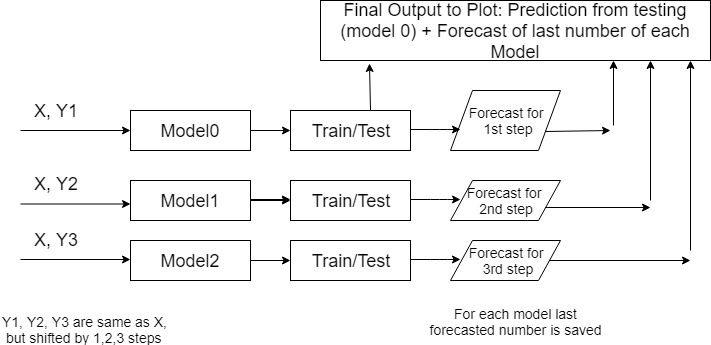Multiple step prediction with separate neural networks

Here we have 3 LSTM models that are getting same X input data but different target Y data. The target data is shifted by number of steps. If model is forecasting the data stock price for day 2 then Y is shifted by 2 elements.
This happens in the following line when i=1:

```yt_ = yt.shift (-i - 1  )
```

The data were obtained from stock prices from Internet.

The number of unit was obtained by running several variations and chosen based on MSE as following:

```
if i==0:
units=20
batch_size=1
if i==1:
units=15
batch_size=1
if i==2:
units=80
batch_size=1
```

If you want run more than 3 steps / models you will need to add parameters to the above code. Additionally you will need add model initialization code shown below.

Each LSTM network was constructed as following:

```
if i == 0 :
fit0 = Sequential ()
fit0.add (LSTM (  units , activation = 'tanh', inner_activation = 'hard_sigmoid' , input_shape =(len(cols), 1) ))
fit0.add(Dropout(0.2))
fit0.add (Dense (output_dim =1, activation = 'linear'))
fit0.compile (loss ="mean_squared_error" , optimizer = "adam")

fit0.fit (x_train, y_train, batch_size =batch_size, nb_epoch =25, shuffle = False)
train_mse[i] = fit0.evaluate (x_train, y_train, batch_size =batch_size)
test_mse[i] = fit0.evaluate (x_test, y_test, batch_size =batch_size)
pred = fit0.predict (x_test)
pred = scaler_y.inverse_transform (np. array (pred). reshape ((len( pred), 1)))
# below is just fo i == 0
for j in range (len(pred)) :
prediction_data[j] = pred[j]
```

For each model the code is saving last forecasted number.
Additionally at step i=0 predicted data is saved for comparison with actual data:

```prediction_data = np.asarray(prediction_data)
prediction_data = prediction_data.ravel()

# shift back by one step
for j in range (len(prediction_data) - 1 ):
prediction_data[len(prediction_data) - j - 1  ] =  prediction_data[len(prediction_data) - 1 - j - 1]

# combine prediction data from first model and last predicted data from each model
prediction_data = np.append(prediction_data, forecast)
```

The full python source code for time series prediction with LSTM in python is shown here

Data can be found here

### Experiment Results

The LSTM neural network was running with the following performance:

```train_mse
[0.01846262458218137, 0.009637593373373323, 0.0018845983509225203]
test_mse
[0.01648362025879952, 0.026161141224167357, 0.01774421124347165]
```

Below is the graph of actual data vs data testing data, including last 3 stock data prices from each model.Multiple step prediction – actual data vs predictions

Accuracy of prediction 98% calculated for last 3 data stock prices (one from each model).

The experiment confirmed that using models (one model for each step) in multistep-ahead time series prediction has advantages. With this method we can adjust parameters of needed LSTM for each step. For example, number of neurons for i=2 was modified to decrease prediction error for this step. And it did not affect predictions for other steps. This is one of machine learning techniques for stock prediction that is described in 

## Prediction Data Stock Prices with Prophet

In the previous post I showed how to use the Prophet for time series analysis with python. I used Prophet for data stock price prediction. But it was used only for one stock and only for next 10 days.

In this post we will select more data and will test how accurate can be prediction data stock prices with Prophet.

We will select 5 stocks and will do prediction stock prices based on their historical data. You will get chance to look at the report how error is distributed across different stocks or number of days in forecast. The summary report will show that we can easy get accuracy as high as 96.8% for stock price prediction with Prophet for 20 days forecast.

### Data and Parameters

The five stocks that we will select are the stocks in the price range between \$20 – \$50. The daily historical data are taken from the web.

For time horizon we will use 20 days. That means that we will save last 20 prices for testing and will not use for forecasting.

### Experiment

For this experiment we use python script with the main loop that is iterating for each stock. Inside of the loop, Prophet is doing forecast and then error is calculated and saved for each day in forecast:

```   model = Prophet() #instantiate Prophet
model.fit(data);
future_stock_data = model.make_future_dataframe(periods=steps_ahead, freq = 'd')
forecast_data = model.predict(future_stock_data)

step_count=0
# save actual data
for index, row in data_test.iterrows():

results[ind][step_count] = row['y']
results[ind][step_count] = row['ds']
step_count=step_count + 1

# save predicted data and calculate error
count_index = 0
for index, row in forecast_data.iterrows():
if count_index >= len(data)  :

step_count= count_index - len(data)
results[ind][step_count] = row['yhat']
results[ind][step_count] = results[ind][step_count] -  results[ind][step_count]
results[ind][step_count] = 100 * results[ind][step_count] / results[ind][step_count]

count_index=count_index + 1
```

Later on (as shown in the above python source code) we count error as difference between actual closed price and predicted. Also error is calculated in % for each day for each stock using the formula:

Error(%) = 100*(Actual-Predicted)/Actual

Results
The detailed result report can be found here. In this report you can see how error is distributed across different days. You can note that there is no significant increase in error with 20 days of forecast and the error always has the same sign for all 20 days.

Below is the summary of error and accuracy for our selected 5 stocks. Also added the column the year of starting point of data range that was used for forecast. It turn out that all 5 stocks have different historical data range. The shortest data range was starting in the middle of 2016.prediction data stock prices with Prophet summary of results

Overall results for accuracy are not great. Only one stock got good accuracy 96.8%.
Accuracy was varying for different stocks. To investigate variation I plot graph of accuracy and beginning year of historical data. The plot is shown below. Looks like there is a correlation between data range used for forecast and accuracy. This makes sense – as the data in the further past may be do more harm than good.

Looking at the plot below we see that the shortest historical range (just about for one year) showed the best accuracy.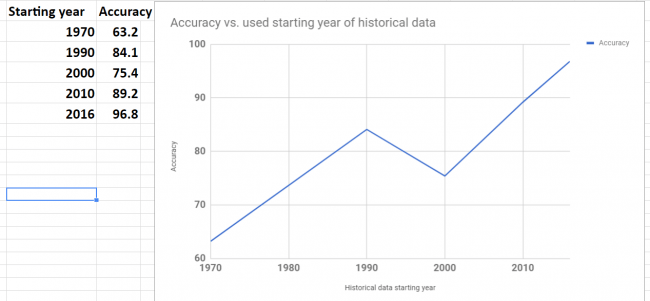prediction data stock prices with Prophet – accuracy vs used data range

Conclusion
We did not get good results (except one stock) in our experiments but we got the knowledge about possible range and distribution of error over the different stocks and time horizon (up to 20 days). Also it looks promising to try do forecast with different historical data range to check how it will affect performance. It would be interesting to see if the best accuracy that we got for one stock can be achieved for other stocks too.

I hope you enjoyed this post about using Prophet for prediction data stock prices. If you have any tips or anything else to add, please leave a comment in the reply box below.

Here is the script for stock data forecasting with python using Prophet.

```import pandas as pd
from fbprophet import Prophet

steps_ahead = 20
fname_path="C:\\Users\\stock data folder"

fnames=['fn1.csv','fn2.csv', 'fn3.csv', 'fn4.csv', 'fn5.csv']
# output fields: actual, predicted, error, error in %, date
fields_number = 6

results= [[[0 for i in range(len(fnames))] for j in range(steps_ahead)] for k in range(fields_number)]

for ind in range(5):

fname=fname_path + "\\" + fnames[ind]

data = pd.read_csv (fname)

#keep only date and close
#delete Open, High,	Low  , Adj CLose, Volume
data.drop(data.columns[[1, 2, 3,5,6]], axis=1)
data.columns = ['ds', 'y', "", "", "", "", ""]

data_test = data[-steps_ahead:]
print (data_test)
data = data[:-steps_ahead]
print (data)

model = Prophet() #instantiate Prophet
model.fit(data);
future_stock_data = model.make_future_dataframe(periods=steps_ahead, freq = 'd')
forecast_data = model.predict(future_stock_data)
print (forecast_data[['ds', 'yhat', 'yhat_lower', 'yhat_upper']].tail(12))

step_count=0
for index, row in data_test.iterrows():

results[ind][step_count] = row['y']
results[ind][step_count] = row['ds']
step_count=step_count + 1

count_index = 0
for index, row in forecast_data.iterrows():
if count_index >= len(data)  :

step_count= count_index - len(data)
results[ind][step_count] = row['yhat']
results[ind][step_count] = results[ind][step_count] -  results[ind][step_count]
results[ind][step_count] = 100 * results[ind][step_count] / results[ind][step_count]

count_index=count_index + 1

for z in range (5):
for i in range (steps_ahead):
temp=""
for j in range (5):
temp=temp + " " + str(results[z][i][j])
print (temp)

print (z)
```

## 10 New Top Resources on Machine Learning from Around the Web

For this post I put new and most interesting machine learning resources that I recently found on the web. This is the list of useful resources in such areas like stock market forecasting, text mining, deep learning, neural networks and getting data from Twitter. Hope you enjoy the reading.

1. Stock market forecasting with prophet – this post belongs to series of posts about using Prophet which is the tool for producing high quality forecasts for time series data that has multiple seasonality with linear or non-linear growth. You will find here different techniques for stock data forecasting.
Prophet is open source software released by Facebook’s Core Data Science team. It is available for download on CRAN and PyPI.

2. Python For Finance: Algorithmic Trading – Another post about stock data analysis with python. This tutorial introduces you to algorithmic trading, and much more.

3. Recommendation and trend analysis is interesting topic. You can read this post to find out how to improve algorithms: recommendation-engine-for-trending-products-in-python In this post author is proposing new trending products algorithm in order to increase serendipity. This will allow to show to user something the user would not expect, but still could find interesting.

4. Word2Vec word embedding tutorial in Python and TensorFlow This tutorial is covering “Word2Vec” technique. This methodology is used in NLP to efficiently convert words into numeric vectors.

5. Best Practices for Document Classification with Deep Learning In this post you will find review of some best practices how to use deep learning for text classification. From the examples in this post you will discover different type of Convolutional Neural Networks (CNN) architecture.

6. How to Develop a Deep Learning Bag-of-Words Model for Predicting Movie Review Sentiment In this post you will find how to use deep learning model for sentiment analysis. The model is simple feedforward network with fully connected layers.

7. How to Clean Text for Machine Learning with Python – Here you will find great and complete tutorial for text preprocessing with python. Also links to resources for further learning are provided too.

8. Gathering Tweets with Python. This tutorial guides you in setting up a system for collecting Tweets.

9. Twitter Data Mining: A Guide To Big Data Analytics Using Python Here you can also find how to connect to Twitter and extract some tweets.

10. Stream data from Twitter using Python This post will show you how to get all identification information required for connecting to Twitter. Also you will find here how to receive tweets via the stream from Twitter.

## Time Series Prediction with Convolutional Neural Networks and Keras

A convolutional neural network (CNNs) is a type of network that has recently
gained popularity due to its success in classification problems (e.g. image recognition
or time series classification) . One of the working examples how to use Keras CNN for time series can be found at this link. This example allows to predict for single time series and multivariate time series.

Running the code from this link, it was noticed that sometimes the prediction error has very high value, may be because optimizer gets stuck in local minimum. (See Fig1. The error is on axis Y and is very high for run 6) So I updated the script to run several times and then remove results with high error. (See Fig2 The Y axis showing small error values). Here is the summary of all changes:

• Created multiple runs that can allow to filter bad results based on error. Training CNN is running 10 times and for each run error data and some other associated data is saved. Error is calculated as square root of sum if squared errors for last 10 predictions during the training.
• Added also plot to see error over multiple runs.
• In the end of script added one plot that showing errors for each run (See Fig1.) , and another plot showing errors only for runs that did not have high error (See Fig2.).
• Added saving keras model to file for each run and then loading it from file for model that showed best results (min error). See  for more information on saving and loading keras models.
• Added ability to load time series from csv file.Error for all runsFig 1.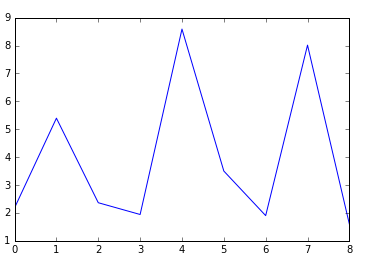Error chart after removing runs with high value errorFig 2.

Below is the full code

``````
#!/usr/bin/env python
"""
This code is based on convolutional neural network model from below link
gist.github.com/jkleint/1d878d0401b28b281eb75016ed29f2ee
"""

from __future__ import print_function, division

import numpy as np
from keras.layers import Convolution1D, Dense, MaxPooling1D, Flatten
from keras.models import Sequential
from keras.models import model_from_json

import matplotlib.pyplot as plt
import csv

__date__ = '2017-06-22'

error_total =[]
result=[]
i=0

def make_timeseries_regressor(window_size, filter_length, nb_input_series=1, nb_outputs=1, nb_filter=4):
""":Return: a Keras Model for predicting the next value in a timeseries given a fixed-size lookback window of previous values.

The model can handle multiple input timeseries (`nb_input_series`) and multiple prediction targets (`nb_outputs`).

:param int window_size: The number of previous timeseries values to use as input features.  Also called lag or lookback.
:param int nb_input_series: The number of input timeseries; 1 for a single timeseries.
The `X` input to ``fit()`` should be an array of shape ``(n_instances, window_size, nb_input_series)``; each instance is
a 2D array of shape ``(window_size, nb_input_series)``.  For example, for `window_size` = 3 and `nb_input_series` = 1 (a
single timeseries), one instance could be ``[, , ]``. See ``make_timeseries_instances()``.
:param int nb_outputs: The output dimension, often equal to the number of inputs.
For each input instance (array with shape ``(window_size, nb_input_series)``), the output is a vector of size `nb_outputs`,
usually the value(s) predicted to come after the last value in that input instance, i.e., the next value
in the sequence. The `y` input to ``fit()`` should be an array of shape ``(n_instances, nb_outputs)``.
:param int filter_length: the size (along the `window_size` dimension) of the sliding window that gets convolved with
each position along each instance. The difference between 1D and 2D convolution is that a 1D filter's "height" is fixed
to the number of input timeseries (its "width" being `filter_length`), and it can only slide along the window
dimension.  This is useful as generally the input timeseries have no spatial/ordinal relationship, so it's not
meaningful to look for patterns that are invariant with respect to subsets of the timeseries.
:param int nb_filter: The number of different filters to learn (roughly, input patterns to recognize).
"""
model = Sequential((
# The first conv layer learns `nb_filter` filters (aka kernels), each of size ``(filter_length, nb_input_series)``.
# Its output will have shape (None, window_size - filter_length + 1, nb_filter), i.e., for each position in
# the input timeseries, the activation of each filter at that position.
Convolution1D(nb_filter=nb_filter, filter_length=filter_length, activation='relu', input_shape=(window_size, nb_input_series)),
MaxPooling1D(),     # Downsample the output of convolution by 2X.
Convolution1D(nb_filter=nb_filter, filter_length=filter_length, activation='relu'),
MaxPooling1D(),
Flatten(),
Dense(nb_outputs, activation='linear'),     # For binary classification, change the activation to 'sigmoid'
))
model.compile(loss='mse', optimizer='adam', metrics=['mae'])
# To perform (binary) classification instead:
# model.compile(loss='binary_crossentropy', optimizer='adam', metrics=['binary_accuracy'])
return model

def make_timeseries_instances(timeseries, window_size):
"""Make input features and prediction targets from a `timeseries` for use in machine learning.

:return: A tuple of `(X, y, q)`.  `X` are the inputs to a predictor, a 3D ndarray with shape
``(timeseries.shape - window_size, window_size, timeseries.shape or 1)``.  For each row of `X`, the
corresponding row of `y` is the next value in the timeseries.  The `q` or query is the last instance, what you would use
to predict a hypothetical next (unprovided) value in the `timeseries`.
:param ndarray timeseries: Either a simple vector, or a matrix of shape ``(timestep, series_num)``, i.e., time is axis 0 (the
row) and the series is axis 1 (the column).
:param int window_size: The number of samples to use as input prediction features (also called the lag or lookback).
"""
timeseries = np.asarray(timeseries)
assert 0 < window_size < timeseries.shape
X = np.atleast_3d(np.array([timeseries[start:start + window_size] for start in range(0, timeseries.shape - window_size)]))
y = timeseries[window_size:]
q = np.atleast_3d([timeseries[-window_size:]])
return X, y, q

def evaluate_timeseries(timeseries, window_size):
"""Create a 1D CNN regressor to predict the next value in a `timeseries` using the preceding `window_size` elements
as input features and evaluate its performance.

:param ndarray timeseries: Timeseries data with time increasing down the rows (the leading dimension/axis).
:param int window_size: The number of previous timeseries values to use to predict the next.
"""
filter_length = 5
nb_filter = 4
timeseries = np.atleast_2d(timeseries)
if timeseries.shape == 1:
timeseries = timeseries.T       # Convert 1D vectors to 2D column vectors

nb_samples, nb_series = timeseries.shape
print('\n\nTimeseries ({} samples by {} series):\n'.format(nb_samples, nb_series), timeseries)
model = make_timeseries_regressor(window_size=window_size, filter_length=filter_length, nb_input_series=nb_series, nb_outputs=nb_series, nb_filter=nb_filter)
print('\n\nModel with input size {}, output size {}, {} conv filters of length {}'.format(model.input_shape, model.output_shape, nb_filter, filter_length))
model.summary()

error=[]

X, y, q = make_timeseries_instances(timeseries, window_size)
print('\n\nInput features:', X, '\n\nOutput labels:', y, '\n\nQuery vector:', q, sep='\n')
test_size = int(0.01 * nb_samples)           # In real life you'd want to use 0.2 - 0.5
X_train, X_test, y_train, y_test = X[:-test_size], X[-test_size:], y[:-test_size], y[-test_size:]
model.fit(X_train, y_train, nb_epoch=25, batch_size=2, validation_data=(X_test, y_test))

# serialize model to JSON
model_json = model.to_json()
with open("model"+str(i)+".json", "w") as json_file:
json_file.write(model_json)
# serialize weights to HDF5
model.save_weights("model"+str(i)+".h5")
print("Saved model to disk")
global i
i=i+1

pred = model.predict(X_test)
print('\n\nactual', 'predicted', sep='\t')
error_curr=0
for actual, predicted in zip(y_test, pred.squeeze()):
print(actual.squeeze(), predicted, sep='\t')
tmp = actual-predicted
sum_squared = np.dot(tmp.T , tmp)
error.append ( np.sqrt(sum_squared) )
error_curr=error_curr+ np.sqrt(sum_squared)
print('next', model.predict(q).squeeze(), sep='\t')
result.append  (model.predict(q).squeeze())
error_total.append (error_curr)
print (error)

def read_file(fn):
'''
Reads the CSV file
-----
RETURNS:
A matrix with the file contents
'''

vals = []
with open(fn, 'r') as csvfile:
tsdata = csv.reader(csvfile, delimiter=',')
for row in tsdata:
vals.append(row)

# removing title row
vals = vals[1:]
y = np.array(vals).astype(np.float)
return y

def main():
"""Prepare input data, build model, eval uate."""
np.set_printoptions(threshold=25)
ts_length = 1000
window_size = 50
number_of_runs=10
error_max=200

print('\nSimple single timeseries vector prediction')
timeseries = np.arange(ts_length)                   # The timeseries f(t) = t
# enable below line to run this time series
#evaluate_timeseries(timeseries, window_size)

print('\nMultiple-input, multiple-output prediction')
timeseries = np.array([np.arange(ts_length), -np.arange(ts_length)]).T      # The timeseries f(t) = [t, -t]
# enable below line to run this time series
##evaluate_timeseries(timeseries, window_size)

print('\nMultiple-input, multiple-output prediction')
timeseries = np.array([np.arange(ts_length), -np.arange(ts_length), 2000-np.arange(ts_length)]).T      # The timeseries f(t) = [t, -t]
# enable below line to run this time series
#evaluate_timeseries(timeseries, window_size)

timeseries = read_file('ts_input.csv')
print (timeseries)

for i in range(number_of_runs):
evaluate_timeseries(timeseries, window_size)

error_total_new=[]
for i in range(number_of_runs):
if (error_total[i] < error_max):
error_total_new.append (error_total[i])

plt.plot(error_total)
plt.show()
print (result)

plt.plot(error_total_new)
plt.show()
print (result)

best_model=np.asarray(error_total).argmin(axis=0)
print ("best_model="+str(best_model))

json_file = open('model'+str(best_model)+'.json', 'r')
loaded_model_json = json_file.read()
json_file.close()
loaded_model = model_from_json(loaded_model_json)

# load weights into new model
loaded_model.load_weights("model"+str(best_model)+".h5")
print("Loaded model from disk")

if __name__ == '__main__':
main()

``````

## Forecasting Time Series Data with Convolutional Neural Networks

Convolutional neural networks(CNN) is increasingly important concept in computer science and finds more and more applications in different fields. Many posts on the web are about applying convolutional neural networks for image classification as CNN is very useful type of neural networks for image classification. But convolutional neural networks can also be used for applications other than images, such as time series prediction. This post is reviewing existing papers and web resources about applying CNN for forecasting time series data. Some resources also contain python source code.

Deep neural networks opened new opportunities for time series prediction. New types of neural networks such as LSTM (variant of the RNN), CNN were applied for time series forecasting. For example here is the link for predicting time series with LSTM.  You can find here also the code. The code provides nice graph with ability to compare actual data and predicted data. (See figure below, sourced from ) Predictions start at different points of time so you can see and compare performance for several predictions.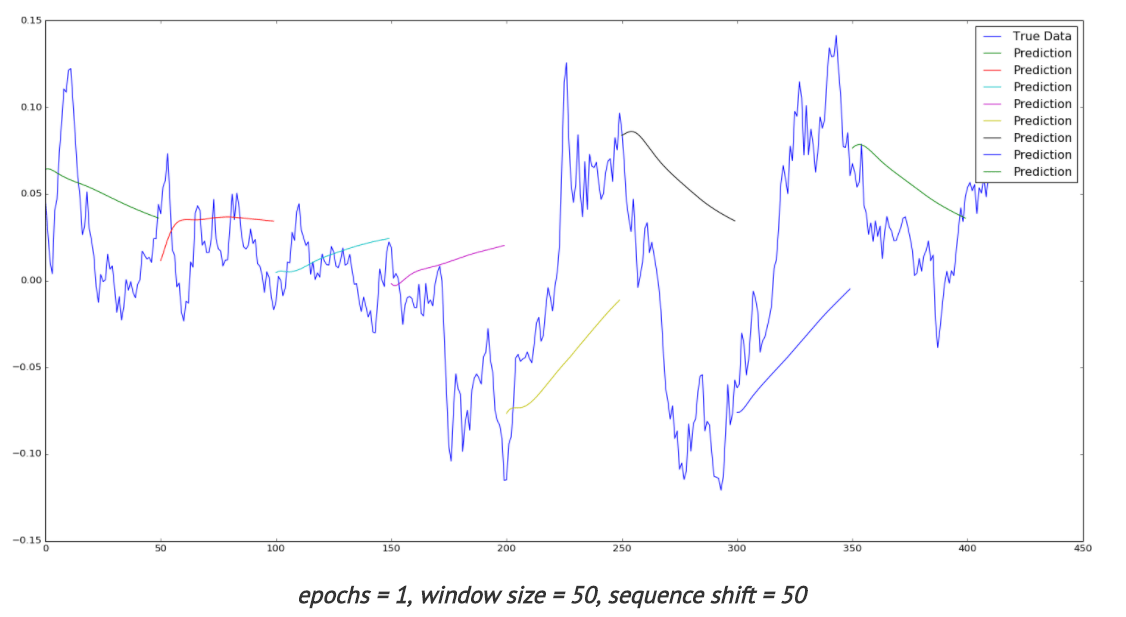Time series with LSTM

Below review is showing different approaches that can be used for forecasting time series data with convolutional neural networks.

1. Raw Data
The simplest way to feed data into neural network is to use raw data. Here is the link  to results of experiments with different types of neural networks including CNN. In this study stock data such as Date,Open,High,Low,Close,Volume,Adj Close were used with 3 types of networks: MLP, CNN and RNN.

CNN architecture was used as 2-layer convolutional neural network (combination of convolution and max-pooling layers) with one fully-connected layer. To improve performance the author suggests using different features (not only scaled time series) like some technical indicators, volume of sales.
According to  it is common to periodically insert a Pooling layer in-between successive Convolution layers in a CNN architecture. Its function is to progressively reduce the spatial size of the representation to reduce the amount of parameters and computation in the network, and hence to also control overfitting. The Pooling Layer operates independently on every depth slice of the input and resizes it spatially, using the MAX operation.

2. Automatic Selection of Features
Transforming data before inputting to neural network is common practice. We can use feature based methods like described here in Feature-selection-time-series-forecasting-python or filtering methods like removing trend, seasonality or low pass / high pass filtering.
With deep learning it is possible to lean features automatically. For example in one research the authors introduce a deep learning framework for multivariate time series classification: Multi-Channels Deep Convolutional Neural Networks (MCDCNN). Multivariate time series are separated into univariate ones and perform feature learning on each univariate series individually. Then a
normal MLP is concatenated at the end of feature learning to do classification. 
The CNN architecture consists of 2 layer CNN (combination of filter, activation and pooling layers) and 2 Fully connected layers that represent classification MLP.

3. Fully Convolutional Neural Network (FCN)
In this study different neural network architectures such as Multilayer Perceptrons, Fully convolutional NN (FCN), Residual Network are proposed. For FCN authors build the final networks by stacking three convolution blocks with the filter sizes {128, 256, 128} in each block. Unlike the MCNN and MC-CNN, any pooling operation is excluded. This strategy helps to prevent overfitting. Batch normalization is applied to speed up the convergence speed and help improve generalization.

After the convolution blocks, the features are fed into a global average pooling layer instead of a fully connected layer, which largely reduces the number of weights. The final label is produced by a softmax layer.  Thus the architecture of neural network consists of three convolution blocks and global average pooling and softmax layers in the end.

4. Different Data Transformations
In this study  CNNs were trained with different data transformations, which included: the entire dataset, spatial clustering, and PCA decomposition. Data was also fit to the hidden modules of a Clockwork Recurrent Neural Network. This type of recurrent network (CRNN) has the advantage of maintaining a high-temporal-resolution memory in its hidden layers after training.

This network also overcomes the problem of the vanishing gradient found in other RNNs by partitioning the neurons in its hidden layers as different ”sub-clocks” that are able to capture the input to the network at different time steps. Here you can find more about CRNN . According to this paper a clockwork RNN architecture is similar to a simple RNN with an input, output and hidden layer. The hidden layer is partitioned into g modules each with its own clock rate. Within each module the neurons are fully interconnected.

5. Analysing Multiple Time Series Relationships
This paper  focuses on analyzing multiple time series relationships such as correlations between them. Authors show that deep learning methods for time series processing are comparable to the other approaches and have wide opportunities for further improvement. Range of methods is discussed and code optimisations is applied for the convolutional neural network for the forecasting time series data domain.

6. Data Augmentation
In this study two approaches are proposed to artificially increase the size of training sets. The first one is based on data-augmentation techniques. The second one consists in mixing different training sets and learning the network in a semi-supervised way. The authors show that these two approaches improve the overall classification performance.

7. Encoding data as image
Another methodology for time series with convolutional neural networks that got popular with deep learning is encoding data as the image. Here data is encoded as images which feed to neural network. This enables the use of techniques from computer vision for classification.
Here  is the link where python script can be found for encoding data as image. It encodes data into formats such as GAF, MTF. The script has the dependencies on python modules such as Numpy, Pandas, Matplolib and Cpickle.

Theory of using image encoded data is described in . In this paper a novel framework proposes to encode time series data as different types of images, namely, Gramian Angular Fields (GAF) and Markov Transition Fields (MTF).

Learning Traffic as Images:
This paper  proposes a convolutional neural network (CNN)-based method that learns traffic as images and predicts large-scale, network-wide traffic speed with a high accuracy. Spatiotemporal traffic dynamics are converted to images describing the time and space relations of traffic flow via a two-dimensional time-space matrix. A CNN is applied to the image following two consecutive steps: abstract traffic feature extraction and network-wide traffic speed prediction. CNN architecture consists of several convolutional and pooling layers and fully connected layer in the end for prediction.

Conclusion

Deep learning and convolutional neural networks created new opportunities for forecasting time series data domain. The above text presents different techniques that can be used in time series prediction with convolutional neural networks. The common part that we can see in most of studies is that feature extraction can be done via deep learning automatically in CNN or in other words, CNNs can learn features on their own. Below you can see architecture of CNN at very high level. The actual implementations can vary in different ways, some of them were shown above.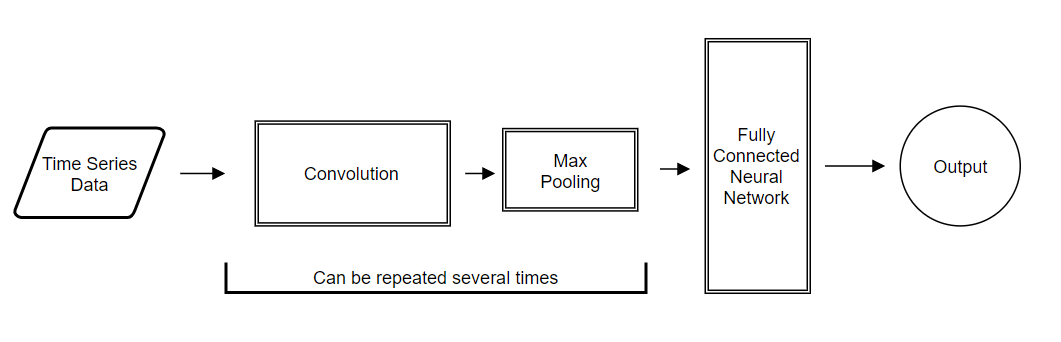CNN Architecture for Forecasting Time Series Data

References